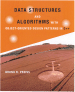Data Structures and Algorithms with Object-Oriented Design Patterns in C++# Sets, Multisets and Partitions

In mathematics a set  is a collection of elements, especially a collection having some feature or features in common. The set may have a finite number of elements, e.g., the set of prime numbers less than 100; or it may have an infinite number of elements, e.g., the set of right triangles. The elements  of a set may be anything at all--from simple integers to arbitrarily complex objects. However, all the elements of a set are distinct--a set may contain only one instance of a given element.

For example,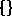,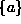,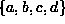and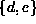are all sets the elements of which are drawn from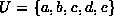. The set of all possible elements, U, is called the universal set . Note also that the elements comprising a given set are not ordered. Thus,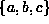and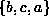are the same set.

There are many possible operations on sets. In this chapter we consider the most common operations for combining sets--union, intersection, difference:

union
The union  (or conjunction ) of sets S and T, written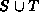, is the set comprised of all the elements of S together with all the element of T. Since a set cannot contain duplicates, if the same item is an element of both S and T, only one instance of that item appears in. If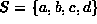and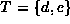, then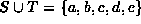.
intersection
The intersection  (or disjunction ) of sets S and T is written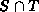. The elements ofare those items which are elements of both S and T. Ifand, then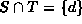.
difference
The difference  (or subtraction ) of sets S and T, written S-T, contains those elements of S which are not also elements of T. I.e., the result S-T is obtained by taking the set S and removing from it those elements which are also found in T. Ifand, then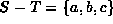.

Figureillustrates the basic set operations using a Venn diagram . A Venn diagram represents the membership of sets by regions of the plane. In Figurethe two sets S and T divide the plane into the four regions labeled I-IV. The following table illustrates the basic set operations by enumerating the regions that comprise each set.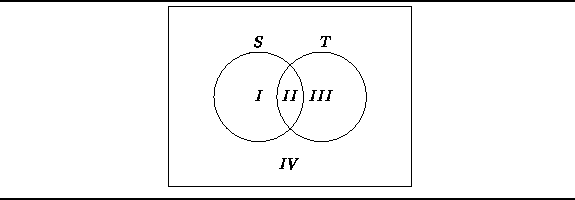Figure: Venn Diagram Illustrating the Basic Set Operations

 set region(s) of FigureU I, II, III, IV S I, II S' III, IV T II, IIII, II, IIIII S-T I T-S IIICopyright © 1997 by Bruno R. Preiss, P.Eng. All rights reserved.